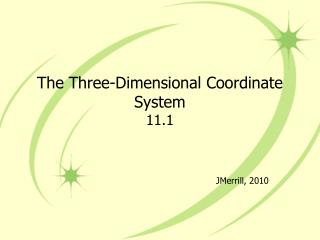DownloadDownload PresentationThe Three-Dimensional Coordinate System 11.1

# The Three-Dimensional Coordinate System 11.1

Download Presentation## The Three-Dimensional Coordinate System 11.1

- - - - - - - - - - - - - - - - - - - - - - - - - - - E N D - - - - - - - - - - - - - - - - - - - - - - - - - - -
##### Presentation Transcript

1. The Three-Dimensional Coordinate System11.1 JMerrill, 2010

2. Solid Analytic Geometry • The Cartesian plane (rectangular coordinate system) is determined by 2 perpendicular number line (x- and y-axis) and their point of intersection (the origin). • To identify a point in space, we need a third dimension. The geometry of this three-dimensional model is called solid analytic geometry. • The 3-D coordinate system is formed by passing a z-axis perpendicular to both the x- and y-axes at the origin.

3. Coordinate Planes Notice we draw the x- and y-axes in the opposite direction X = directed distance from yz-plane to some point P Y= directed distance from xz-plane to some point P Z= directed distance from xy-plane to some point P (x,y,z) So, to plot points you go out, over, up/down

4. Octants • The 3-D system can have either a right-handed or a left-handed orientation. • We’re only using the right-handed orientation meaning that the octants (quadrants) are numbered by rotating counterclockwise around the positive z-axis. • There are 8 octants.

5. Octants

6. Plotting Points in Space • Plot the points: • (2,-3,3) • (-2,6,2) • (1,4,0) • (2,2,-3) Draw a sideways x, then put a perpendicular line through the origin.

7. Formulas • You can use many of the same formulas that you already know because right triangles are still formed.

8. The Distance Formula • It looks the same in space as it did before except with a third coordinate:

9. Example • Find the distance between (1, 0, 2) and (2, 4,-3)

10. Midpoint Formula • The midpoint formula is • What is the midpoint if you make a 100 on a test and an 80 on a test? • So the midpoint is just the average of the x’s, y’s, and z’s.

11. Midpoint You Do • Find the midpoint of the line segment joining (5, -2, 3) and (0, 4, 4)

12. Equation of a Sphere • The equation of a circle is x2 + y2 = r2 • If the center is not at the origin, then the equation is (x-h)2 + (y-k)2 = r2 • The equation of a sphere whose center is at (h,k,j) with radius r is (x-h)2 + (y-k)2 + (z–j)2= r2

13. Finding the Equation of a Sphere • Find the standard equation of a sphere with center (2,4,3) and radius 3 • (x-h)2 + (y-k)2 + (z–j)2= r2 • (x-2)2 + (y-4)2 + (z–3)2 = 32 • Does the sphere intersect the plane? • Yes. The center of the sphere is 3 units above the y-axis and has a radius of 3. It intersects at (2,4,0).

14. Finding the Center and Radius of a Sphere • Find the center and radius of the sphere given by x2 + y2 + z2 – 2x + 4y – 6z +8 = 0 • This works the same way as it did in 2-D space. In order to find the center, we must put the equation into standard form, which means completing the square.

15. Finding the Center and Radius of a Sphere • x2 + y2 + z2 – 2x + 4y – 6z +8 = 0 • (x-1)2 + (y+2)2 + (z-3)2= 6 • The center is (1,-2,3) and the radius is √6.